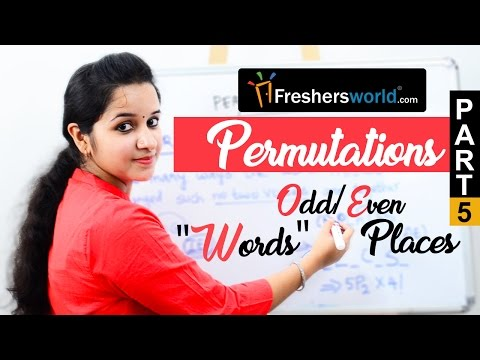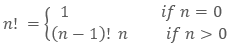# Permutation and Combination

##• The word permutation means the arrangement of the alike or different objects taken some or all at a time. So we can observe the word ‘arrangement’ used in the definition of permutation. Here the arrangement means selection as well as order. That means the order in which the objects are selected has also been taken care of in this case.
• For Example – The number of 5 digit numbers can be formed using the digits 0, 1, 2, 3, 4, and 5.
• In this example, we just do not have to select the 5 digits out of given 6 digits but also have to see the number of possible causes for the different arrangements.  So the numbers 34251, 21034, 42351 are all different cases.

We will try to explore these definitions in the upcoming heading.

1. What is Factorial Notation in mathematics?

• Since the definition or the formula of both, permutation and combination, requires the use of factorial notation, so let’s first understand this here before learning any further.
• In Mathematics, the factorial is represented by the symbol ‘!’ i.e. if we have to write 5 factorial, so it will be written as 5! So in general factorial of any positive number n will be represented by n!.
• Mathematically,where n is any positive integer.

• So, 4! = 3! x 4 = 2! x 3 x 4 = 1! x 2 x 3 x 4 = 0! x 1 x 2 x 3 x 4 = 1 x 2 x 3 x 4
• Similarly we can say for any positive integer ‘n’
• n! = n x (n – 1) x (n – 2) x …….. x 3 x 2 x 1.
• Thus, we seeing the above equation, we may also define the factorial of any positive integer n as ‘the product of all the positive integers less than or equal to n’.
• Just see below for the factorial of few frequently used numbers.
• 0! = 1
• 1! = 1
• 2! = 2 x 1 = 2
• 3! = 3 x 2 x 1 = 6
• 4! = 4 x 3 x 2 x 1 = 24
• 5! = 5 x 4 x 3 x 2 x 1 = 120 and so on.

1. Differentiate Permutation and Combination

The very basic difference in permutation and combination is the order of the objects considered. In combination, the order is not considered at all while for permutation it is a must. So the permutation is the ordered arrangement while the combination is the unordered selection. From the three alphabets A, B, and C, the permutation of these 3 letters will be ABC, ACB, BAC, BCA, CBA, and CAB. While the combination of 3 letters will be just (A, B, C).Permutation answers the number of arrangements while the combination explains the possible number of selections. Permutation of a single combination can be multiple but the combination of a single permutation is unique (considering all at a time).